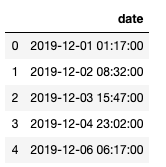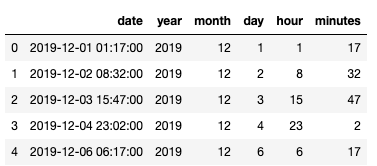Search
•Magdalena Konkiewicz

# Extracting features from dates in pandasPhoto by Brooke Lark on Unsplash

Once you start working with pandas you will notice that dates have their own data type. This is very handy, you can use it to sort cells according to dates and is important for time series analysis and finding trends in data. The date itself actually conveys much more information that you can think of just by looking at the simple timestamp.

In this article, you will learn how to extract all possible features from data objects in pandas. Each feature type extraction will be discussed in detail with example code. After reading this you will be able to extract the following information:

• year

• month

• day

• hour

• minute

• day of year

• week of year

• day of week

• quarter

Let’s get started and create some data time objects in pandas. We can do it using pandas date_range function in the following way.

```
import datetime
import numpy as np
import pandas as pd

dates = pd.Series(pd.date_range('2019-12-01 1:17', freq='31H15min',  periods=5))
df = pd.DataFrame(dict(date=dates))

```As you can see we have created a data frame with one column called date and we filled it in with five different timestamps. The first timestamp is 12 of January 2019 at 1: 17 AM and the following timestamps are incremented by 31h and 15 minutes using the freq parameter.

This will be enough to demonstrate some simple feature extraction so let’s get into it.

Getting year feature

We will start by getting the year feature. This is simple enough, we just have to call dt.year on the date column. Note that I will be saving the result as a new column in the data frame.

```
df['year'] = df.date.dt.year

```You can see that all year feature got saved in the column ‘year’ and for all rows, it is equal to 2019.

Getting month feature

In a similar manner, we will get a month for each row now.

```
df['month'] = df.date.dt.month

```Getting day feature

Let’s now get the day of the month now.

```
df['day'] = df.date.dt.day

```Getting hour feature

And now let’s get an hour from the date.

```
df['hour'] = df.date.dt.hour

```Getting minute feature

And let’s get the minute feature.

```
df['minutes'] = df.date.dt.minute

```Getting day of the year feature

Let’s now get the day of the year feature. Note that this is the ordinal day of the year and is different from the day of the month feature we have extracted before.

```
df['day_of_year'] = df.date.dt.dayofyear

```Getting week of the year feature

Another important feature that we can extract is the week of the year.

```
df['day_of_year'] = df.date.dt.dayofyear

```Getting day of the week feature

Getting day of the week feature is probably my favourite feature to extract from data as it allows you for weekly analysis and a lot of data will have weekly patterns.

```
df['day_of_week'] = df.date.dt.dayofweek
df['day_of_week_name'] = df.date.dt.weekday_name

```We have created two columns day_of_week where the weekdays are denoted with numbers (Monday=0 and Sunday=6) and day_of_week_name column where the days are represented by its weekday name in the form of strings. I like to have them in both forms as the numerical form makes the data ready for machine learning modelling and the string form looks nice on the graphs when we analyze the data.

Also, note that when calling dt.weekday_name on the date it is spelt with an underscore, unlike other features that we were extracting.

Getting a quarter feature

The last feature that we will extract is a quarter of the year.

```
df['quarter'] = df.date.dt.quarter

```Getting even more features

Above we have covered basic features that you can extract from pandas date objects. I have focused on the most common features but there are even more features that you could extract depending on your needs.

• is the day a weekday or weekend,

• is the year a leap year or not,

• seconds, microseconds and nanoseconds

• and more.

Summary

In this article, you have learnt how to get basic features from the date object in pandas that you can use for exploratory data analysis and modelling. It’s time to use it on real data set now, so happy coding!